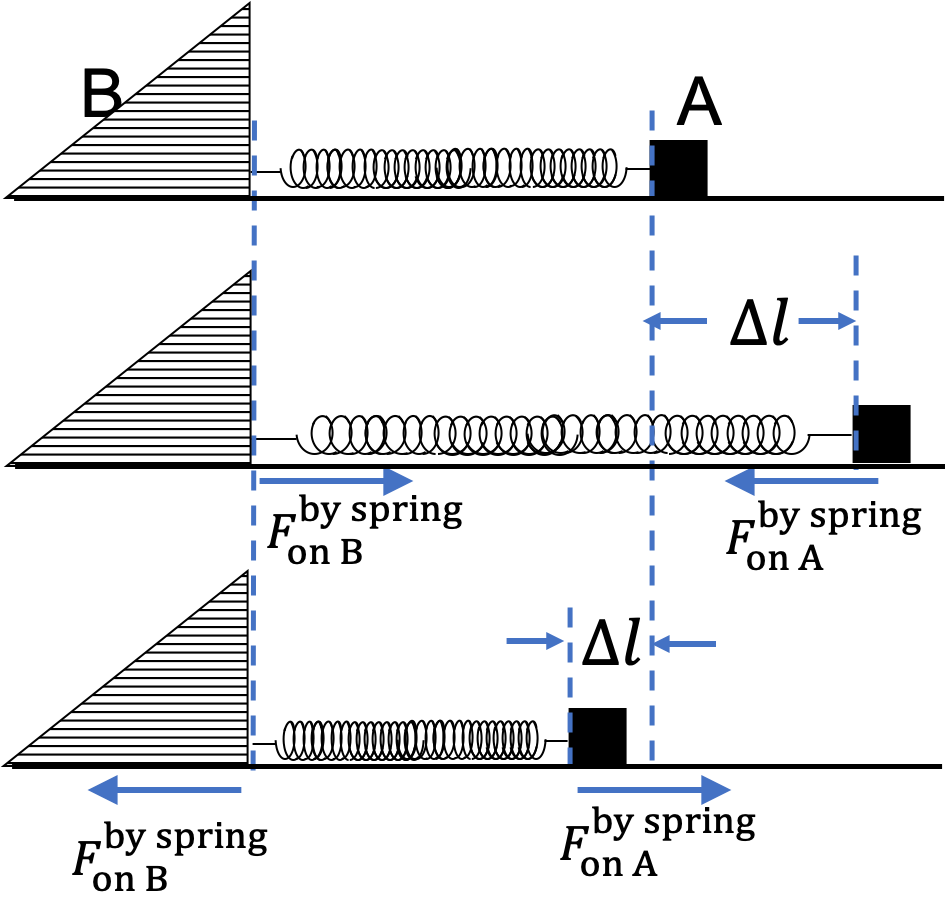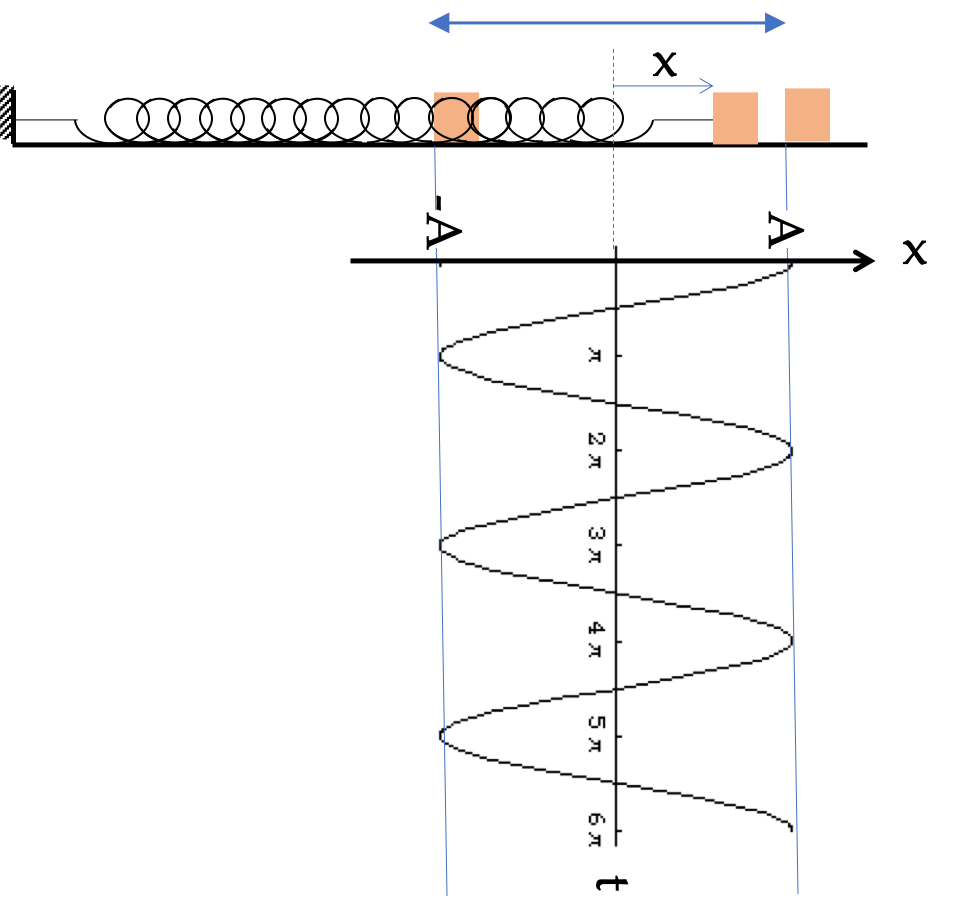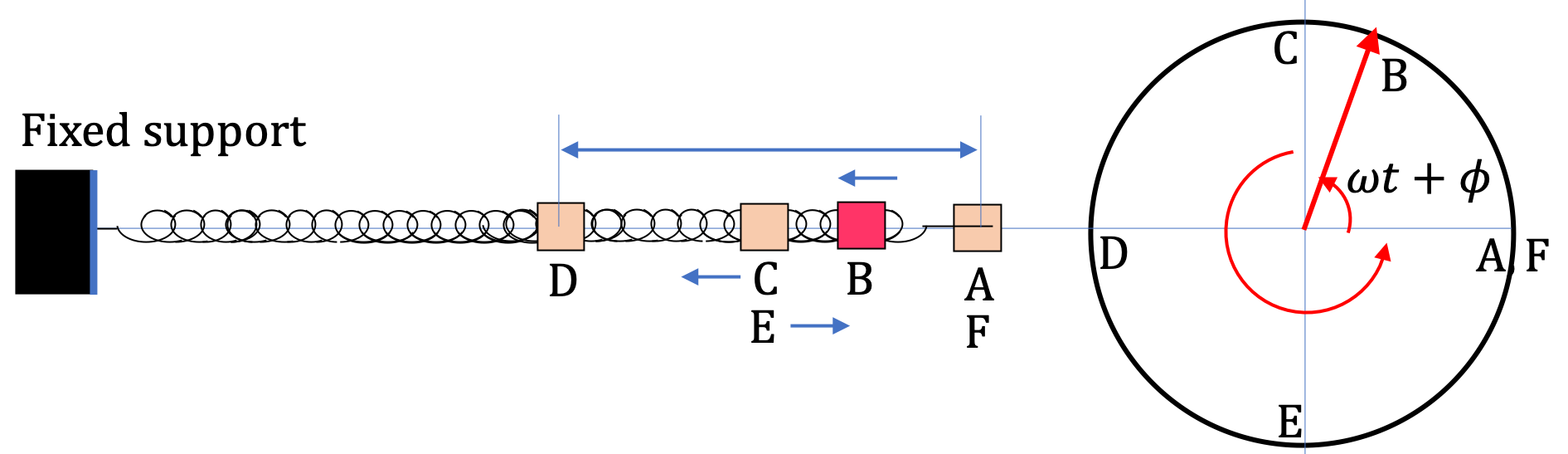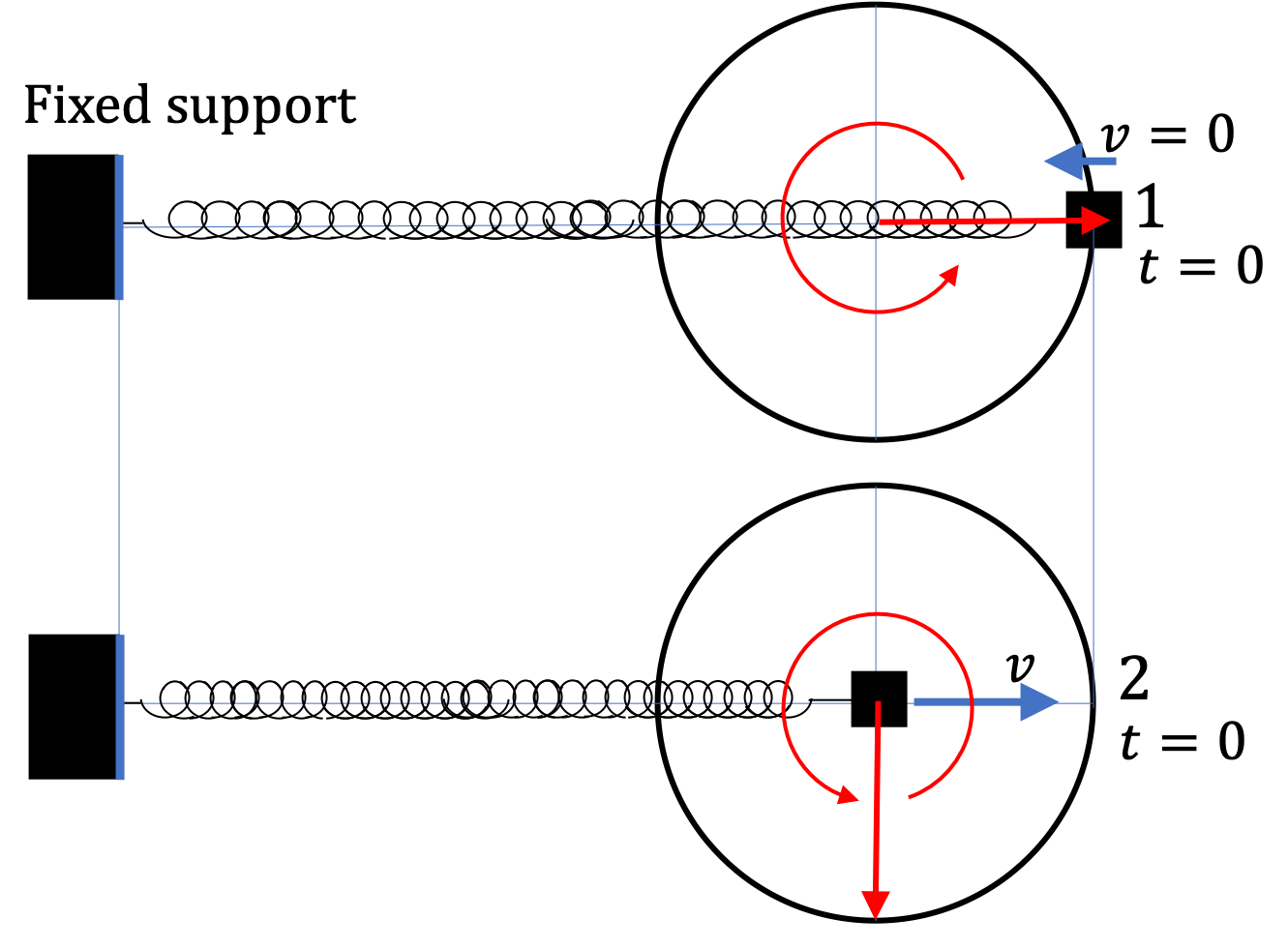## Section13.2Simple Harmonic Oscillator

Oscillatory motions are ubiquitous is nature, from the to and fro swaying of high-rise buildings to back and forth motion of pendulums, to vibrations of atoms in molecules, vibrations of guitar string, just to name a few.

A block attached to a spring provides an ideal model to study harmonic oscillations. We often call this model system a simple harmonic oscillator (SHO). In real blocks attached to spring, friction and drag forces take away energy and damp the motion of the block, eventually stopping it. But, in this section, we will not worry about these effects - as a result, we will get a perpetual motion - the block will be oscillating forever! In a later section we will address damping as well as how additional energy can be put into the block to keep it going.

Consider a block A of mass $m$ attached to a spring of spring constant $k$ placed on a horizontal frictionless surface. The other end of the spring is attached to an infinite-mass immovable support B.

The spring has a natural length, which we can call its equilibrium length. When spring is extended or shortened, the spring pulls in or pushes out at the two ends by a force, whose magnitude $F_{s}$ is equal to the amount $\Delta l$ by which the length is different from the equilibrium length.

\begin{equation} F = k\,\Delta l.\label{eq-harmonic-scillator-spring-force-general}\tag{13.2.1} \end{equation}The direction of this force is from the current location of the end of the spring to the point at which the spring has the natural length. Another way to look at the spring force is that the force between objects at the two ends of the spring is attractive when the spring is stretched and repulsive when the spring is compressed.

If the block of mass $m$ is started with displacement $x=x_0$ and velocity $v_x = v_0\text{,}$ the resulting motion is an oscillatory motion with $x$ between $x=-A$ and $x=+A$ with

\begin{equation} A = \sqrt{ x_0^2 + \left( v_0/\omega \right)^2 },\tag{13.2.2} \end{equation}

with period

\begin{equation} T = 2\pi \sqrt{ \dfrac{m}{k} }.\tag{13.2.3} \end{equation}

Qunatity $A$ is called amplitude of the motion.

Astronauts must keep a good record of their masses. Th normal bathroom scales that use gravity to get mass would not work in space. Instead, mass can be obtained from $T = 2\pi\sqrt{m/k}$ of a spring/mass oscillations. In this method, we find the mass of an astronaut by strapping the astronaut to a chair which is attached to a spring, and observing the motion.

The mass of the chair is $200\text{ kg}\text{.}$ When astronaut is not in the chair, the period of oscillation is $1.50\text{ sec}\text{.}$ The period changes to $1.75\text{ sec}\text{.}$

What is the mass of the astronaut?

Hint

From the motion of the chair alone, find the $k$ of the spring. Or, use $T\propto\sqrt{m}\text{.}$

$72.2\text{ kg}\text{.}$

Solution

Let $M$ be the mass of the astronaut. Thinking of $T = 2\pi\sqrt{m/k}$ as $T\propto\sqrt{m}\text{,}$ gives us the following ratio

\begin{equation*} \dfrac{T_1}{T_2} = \sqrt{ \dfrac{M_1}{M_2} }\ \ \Longrightarrow\ \ M_2 = \left( \dfrac{T_2}{T_1} \right)^2\, M_1. \end{equation*}

Setting $M_1 = 200\text{,}$ $M_2 = M + 200\text{,}$ $T_1=1.50$ and $T_2=1.75$ we have

\begin{equation*} M = \left( \dfrac{1.75}{1.50} \right)^2 \times 200 - 200 = 72.2\text{ kg}. \end{equation*}

### Subsection13.2.1Equation of Motion and Solution

To study the block analytically, we choose $x$ coordinate system that has a positive $x$ axis pointed in the direction in which the spring stretches and we place the origin at the place where the block is located when spring has length equal to the equilibrium length, i.e., when there is zero force on the block.Figure 13.2.2. Using coordinates to express the spring force analytically, $F_x = - k x\text{.}$

Let $x$ denote the $x$ coordinate of the block at instant $t \text{.}$ With the arrangement in Figure 13.2.2, when the block's position has $x \gt 0 \text{,}$ the force on the block is pointed left, i.e., $F_x \lt 0\text{,}$ and when the block's position has $x \lt 0\text{,}$ we have $F_x \gt 0\text{,}$ where $F_x$ is the $x$ component of the force vector $\vec F$ as usual. Therefore, force by spring has $x$-component given by

\begin{equation} F_x = -k\, x.\tag{13.2.4} \end{equation}

Therefore, $x$-equation of motion of the block is

\begin{equation} m a_x = - kx.\label{eq-harmonic-scillator-spring-force-kx}\tag{13.2.5} \end{equation}

We would like to get a formula that tells us $x(t)$ for given initial position and velocity, $x_0$ and $v_0\text{.}$ This is called solving the equation of motion. If you solve Eq. (13.2.5) for acceleration, they don't take you anywhere.

\begin{equation*} a_x = -\dfrac{k}{m}\, x\ \ \ \text{or}\ \ \ x = -\dfrac{m}{k}\, a_x, \end{equation*}

You need to think of Eq. (13.2.5) as a differential equation and solve that differential equation. We will not do that here. We will just borrow the solution from the folks who have solved this equation.

The solution is more compactly written if we replace $k/m$ by another symbol, actually, the square of a symbol $\omega^2\text{,}$ since we want to make sure this number is always positive.

\begin{equation} \omega^2 = \sqrt{\dfrac{k}{m}}.\tag{13.2.6} \end{equation}

Then, position of the block that starts at $x=x_0$ and $x$ component of the velocity $v=v_0$ is completely determined by

\begin{equation} x(t) = x_0\, \cos(\omega t) + \dfrac{v_0}{\omega}\sin(\omega t).\label{eq-harmonic-scillator-x-in-x0-v0}\tag{13.2.7} \end{equation}

The oscillator moves about the origin symmetrically. The largest $x$ away from the equilibrium is called the amplitude, which is obtained by combining the coefficients of the cosine and sine terms as follows. The amplitude is usually denoted by $A\text{.}$

\begin{equation} A = \sqrt{ x_0^2 + \left( v_0/\omega \right)^2 }.\tag{13.2.8} \end{equation}

The velocity at intstant $t$ is given by

\begin{equation} v_x(t) = v_0\, \cos(\omega t) - \omega\,x_0\, \sin(\omega t),\label{eq-harmonic-scillator-v-in-x0-v0}\tag{13.2.9} \end{equation}

from which we get the largest speed in the motion to be

\begin{equation} \text{Largest speed } = \sqrt{v_0^2 + \left(\omega^2 x_0^2\right)^2 },\tag{13.2.10} \end{equation}

which occurs when the block passes through the equilibrum position, i.e., $x=0\text{.}$

Contrast these formulas for $x$ and $v$ to the constant acceleration motion for acceleration $a_x \text{.}$

\begin{align*} \text{Constant}\amp \text{ Acceleration Motion:}\\ \amp x(t) = x_0 + v_0)\, t + \dfrac{1}{2}\, a_x\, t^2, \\ \amp v(t) = v_0 + a_x\, t. \end{align*}

We don't have this situation here. The acceleration of a simple harmonic oscillator oscialltes in time and is definitely not constant.

### Subsection13.2.2Multiple Ways of Writing the Solution

The solution in Eq. (13.2.7) is good for some purposes but not convenient for some other purposes. For instance, if we wanted to know the furthest from the equilibrium the block will go, its not $x_0\text{,}$ and it is not clear right away how we would get the answer. There is another way to write a sum of cosine and sine of the same argument using the trig formulas for sum of angles.

For instance, we can write Eq. (13.2.7), which we now refer to as two-amplitudes form, also in the following form, which we will call amplitude-phase form.

\begin{equation} x(t) = A\,\cos(\omega t + \phi),\label{eq-harmonic-scillator-x-in-Acosphi}\tag{13.2.11} \end{equation}

where $A$ is the amplitude and $\phi$ the phase constant. Then, the velocity is

\begin{equation} v(t) = -\omega A\,\sin(\omega t + \phi),\label{eq-harmonic-scillator-v-in-Acosphi}\tag{13.2.12} \end{equation}

Another way to write in amplitude-phase form is as a sine function.

\begin{equation} x(t) = B\,\sin(\omega t + \phi_s),\label{eq-harmonic-scillator-x-in-Bsintheta}\tag{13.2.13} \end{equation}

Our convention is to write as positive amplitude times a cosine and use the following to convert anything written in sine to cosine, which makes writing in sine superflous.

\begin{equation} \sin(\theta) = \cos(\theta - \pi/2).\tag{13.2.14} \end{equation}

If we find an expression with negative amplitude times a cosine, we can convert that to positive amplitude times cosine by

\begin{equation} -\cos(\theta) = \cos(\theta - \pi).\tag{13.2.15} \end{equation}

The new constants $A$ and $\phi$ are related to $x_0$ and $v_0$ in Eq. (13.2.7). To find the relation, we expand the right side in this equation,

\begin{equation*} A\,\cos(\omega t + \phi) = A\, \cos\omega t\,\cos\phi - A\, \sin\omega t\,\sin\phi, \end{equation*}

and compare the result to Eq. (13.2.7). This gives the following two equations.

\begin{align*} \amp A\,\cos\phi = x_0,\\ \amp A\,\sin\phi = -\dfrac{v_0}{\omega}. \end{align*}

Squarring and adding these will give us $A^2$ and dividing will give us $\tan\phi\text{.}$ We take the notation that $A$ be positive.

\begin{align*} \amp A = \sqrt{ x_0^2 + \dfrac{v_0^2}{\omega^2} },\\ \amp \tan\phi = -\dfrac{v_0}{\omega x_0}. \end{align*}

The velocity corresponding to the form (13.2.11) is

\begin{equation} v_x(t) = -\omega\, A\,\sin(\omega t + \phi).\label{eq-harmonic-scillator-vx-in-omegaAcosphi}\tag{13.2.16} \end{equation}

This says that the velocity oscillates between $-\omega A$ and $\omega A\text{.}$ Clearly, oscillator has turns in its motion, where velocity must be zero. Turning point at $x=+A$ and $x=-A$ correspond to $v=0\text{.}$

A harmonic oscillator oscillates on $x$ axis about the origin with its $x$ coordinate given by

\begin{equation*} x = 3\, \cos\,\left( 15\, t \right) + 4\, \sin\,\left( 15\, t \right) \end{equation*}

where $t$ is in seconds and $x$ in $\text{cm}\text{.}$

(a) What is the amplitude of the oscillations?

(b) What is the angular frequency of the oscillator?

(c) How many cycles of oscillations occurs in $5\text{ sec}\text{?}$

(d) What is the period of the oscillator?

(e) Where is the oscillator at $t = 0\text{?}$

(f) How fast is the oscillator moving at $t = 0$ and which way? Note: from the formula of $x\text{,}$ the $x$ component of velocity is

\begin{equation*} v = -45\, \sin\,\left( 15\, t\right) + 60\, \cos\,\left( 15\, t \right). \end{equation*}

If you have Calculus background, try deriving this yourself.

Hint

Use two-amplitiudes form of the solution in the text.

(a) $5\text{ cm}\text{,}$ (b) $15\text{ sec}^{-1}\text{,}$ (c) $11.94\text{ cycles}\text{,}$ (d) $0.42\text{ sec} \text{,}$ (e) $3\text{ cm}\text{,}$ (f) $60\text{ cm/sec}$ in the direction of positive $x$ axis.

Solution

(a) From the two-amplitudes form of the solution, we get the amplitude immediately.

\begin{equation*} \text{Amplitude } = \sqrt{ (3\text{ cm})^2 + (4\text{ cm})^2 } = 5\text{ cm}. \end{equation*}

(b) From the argument of cosine or sine, here, we see how the periodic motion varies in time. Equating the time part to $\omega t$ we immediately get $\omega\text{.}$

\begin{equation*} \omega = 15\text{ sec}^{-1}. \end{equation*}

(c) First we find the frequency from $\omega\text{.}$

\begin{equation*} f = \dfrac{\omega}{2\pi} = \dfrac{15}{2\pi} = 2.387\text{ Hz}. \end{equation*}

That is, 2.387 cycles each second. Therefore, we will have

\begin{equation*} N = 2.387\text{ Hz} \times 5\text{ sec} = 11.94\text{ cycles}. \end{equation*}

(d) Inverting the frequency gives the period.

\begin{equation*} T = \dfrac{1}{f} = \dfrac{1}{2.387\text{ Hz}} = 0.42\text{ sec}. \end{equation*}

(e) Set $t=0$ in the given $x$ to get

\begin{equation*} x_0 = 3\text{ cm}. \end{equation*}

(f) Set $t=0$ in the given $v$ to get

\begin{equation*} v_0 = 60\text{ cm/sec}. \end{equation*}

Since $v_0$ is positive, the block is moving in the direction of positive $x$ axis.

A harmonic oscillator oscillates on $x$ axis about the origin with its $x$ coordinate given by

\begin{equation*} x = 10\, \cos\,\left( 15\, t + \dfrac{\pi}{4}\right), \end{equation*}

where $t$ is in seconds and $x$ in $\text{cm}\text{.}$

(a) What is the amplitude of the oscillations?

(b) What is the angular frequency of the oscillator?

(c) How many cycles of oscillations occurs in $5\text{ sec}\text{?}$

(d) What is the period of the oscillator?

(e) Where is the oscillator at $t = 0\text{?}$

(f) How fast is the oscillator moving at $t = 0$ and which way? Note: from the formula of $x\text{,}$ the $x$ component of velocity in $\text{cm/s}$ is

\begin{equation*} v = -150\, \sin\,\left( 15\, t + \dfrac{\pi}{4}\right). \end{equation*}

If you have Calculus background, try deriving this yourself.

Hint

For all parts: consult amplitude/phase form of the solution.

(a)$10\text{ cm}\text{,}$ (b)$15\text{ sec}^{-1}\text{,}$ (c)$11.94\text{ cycles}\text{,}$ (d)$0.42\text{ sec} \text{,}$ (e)$7.07\text{ cm}\text{,}$ (f)$106\text{ cm/sec}$ towards the origin.

Solution

(a) From the amplitude/phase form of the solution, we get the amplitude immediately.

\begin{equation*} \text{Amplitude } = 10\text{ cm}. \end{equation*}

(b) From the argument of cosine, here, we see how the periodic motion varies in time. Equating the time part to $\omega t$ we immediately get $\omega\text{.}$

\begin{equation*} \omega = 15\text{ sec}^{-1}. \end{equation*}

(c) First we find the frequency from $\omega\text{.}$

\begin{equation*} f = \dfrac{\omega}{2\pi} = \dfrac{15}{2\pi} = 2.387\text{ Hz}. \end{equation*}

That is, 2.387 cycles each second. Therefore, we will have

\begin{equation*} N = 2.387\text{ Hz} \times 5\text{ sec} = 11.94\text{ cycles}. \end{equation*}

(d) Inverting the frequency gives the period.

\begin{equation*} T = \dfrac{1}{f} = \dfrac{1}{2.387\text{ Hz}} = 0.42\text{ sec}. \end{equation*}

(e) Set $t=0$ in the given $x$ to get

\begin{equation*} x_0 = 10\, \cos\,\left( \dfrac{\pi}{4}\right) = 7.07\text{ cm}. \end{equation*}

(f) Set $t=0$ in the given $v$ to get

\begin{equation*} v_0 = -150\, \sin\,\left( \dfrac{\pi}{4}\right) = -106\text{ cm/sec}. \end{equation*}

That is, it has speed $106\text{ cm/sec}$ and moving to the left.

The solution of a Simple Harmonic Motion can be written in three ways:

\begin{align*} x(t) \amp = C_1\ \cos(\omega t) + C_2\ \sin(\omega t)\\ \amp = A \cos(\omega t + \phi_c)\\ \amp = B \sin(\omega t + \phi_s) \end{align*}

Find the relations among (a) $C_1\text{,}$ $C_2\text{,}$ $A\text{,}$ $\phi_c\text{,}$ (b) $C_1\text{,}$ $C_2\text{,}$ $B\text{,}$ $\phi_s\text{,}$ and (c) $A\text{,}$ $\phi_c\text{,}$ $B\text{,}$ $\phi_s\text{.}$ Trig formulas $\cos(A+B) = \cos\,A\cos\,B-\sin\,A\sin\,B \text{,}$ $\sin(A+B)=\sin\,A\cos\,B+\sin\,B\cos\,A\text{,}$ and $\sin(\theta + \pi/2) = \cos\,\theta$ will be helpful.

Hint

Expand the amplitude/phase form, and then equate the coefficients of $\cos(\omega t)$ and similarly for $\sin(\omega t)\text{.}$

(a) $A\cos\,\phi_c = C_1\text{,}$ $A\sin\,\phi_c = C_2\text{,}$ $A = \sqrt{ C_1^2 + C_2^2 }\text{,}$ $\tan\,\phi_c = -\dfrac{C_2}{C_1}\text{.}$

(b) $B \sin\,\phi_s = C_1\text{,}$ $B \cos\,\phi_s = C_2\text{,}$ $B = \sqrt{ C_1^2 + C_2^2 }\text{,}$ $\tan\,\phi_s = \dfrac{C_1}{C_2}\text{.}$

(c) $B = A,\ \ \phi_s = \phi_c +\pi/2\text{.}$

Solution 1 (a)

(a) Let us expand $A \cos(\omega t + \phi_c)\text{.}$

\begin{equation*} A \cos(\omega t + \phi_c) = (A\cos\,\phi_c)\, \cos (\omega t) - (A\sin\,\phi_c)\, \sin (\omega t). \end{equation*}

Equate the coefficients of $\cos(\omega t)$ and similarly for $\sin(\omega t)$ in this expression to that in $C_1\ \cos(\omega t) + C_2\ \sin(\omega t)$ we get

\begin{align*} A\cos\,\phi_c \amp = C_1, \\ -A\sin\,\phi_c \amp = C_2. \end{align*}

From this we can get

\begin{align*} \amp A = \sqrt{ C_1^2 + C_2^2 }, \\ \amp \tan\,\phi_c = -\dfrac{C_2}{C_1}. \end{align*}
Solution 2 (b)

(a) Let us expand $B \sin(\omega t + \phi_s)\text{.}$

\begin{equation*} B \sin(\omega t + \phi_s) = (B \cos\,\phi_s)\, \sin (\omega t) + (B\sin\,\phi_s)\, \cos (\omega t). \end{equation*}

Equate the coefficients of $\cos(\omega t)$ and similarly for $\sin(\omega t)$ in this expression to that in $C_1\ \cos(\omega t) + C_2\ \sin(\omega t)$ we get

\begin{align*} B \sin\,\phi_s \amp = C_1, \\ B \cos\,\phi_s \amp = C_2. \end{align*}

From this we can get

\begin{align*} \amp B = \sqrt{ C_1^2 + C_2^2 }, \\ \amp \tan\,\phi_s = \dfrac{C_1}{C_2}. \end{align*}
Solution 3 (c)

(c) Since $\sin(\theta + \pi/2) = \cos\,\theta\text{,}$ we can write both of then in either cosines or sines forms. Let us write the cosine into sine to get

\begin{equation*} A\cos(\omega t + \phi_c) = A\sin(\omega t + \phi_c +\pi/2). \end{equation*}

Equating this to the sine-form given, we immediately get

\begin{equation*} B = A,\ \ \phi_s = \phi_c +\pi/2. \end{equation*}

A block of mass $2\text{ kg}$ is attached to a spring of spring constant $32\text{ N/m}$ and placed on a frictionless surface. The other end of the spring is attached to a fixed support. At $t=0\text{,}$ the block is hit with a hammer such that it instantaneously gains speed of $0.8\text{ m/s}$ and starts to compress the spring. You can assume that at $t=0$ the block is at the equilibrium position.

(a) With $x$ axis along the spring and positive $x$ axis in the direction in which the spring stretches, find the position and velocity of the block at an arbitrary instant $t\text{.}$

(b) What is the amplitude of the oscillations?

Hint

(a) The initial velocity component is negative. (b) Read amplitude from $x(t) \text{.}$

(a) $x(t) = - 0.2\text{ m }\,\sin(4\,t )\text{,}$ $v(t) = - 0.8 \text{ m/s } \,\cos(4\,t )\text{,}$ (b) $0.2\text{ m } \text{.}$

Solution 1 (a)

Since the block at $t=0$ is moving in the opposite direction to the positive $x$ axis, we have the following initial condition on the $x$ component of the velocity

\begin{equation*} v_0 = - 0.8\text{ m/s}. \end{equation*}

The initial position is $x_0 = 0$ since it is at the equilibrium position. From the given mass and spring constant, we get the angular frequency to be

\begin{equation*} \omega = \sqrt{ \dfrac{k}{m} } = 4\text{ sec}^{-1}. \end{equation*}

Using these in the two-amplitudes-form of the solution we get

\begin{align*} \amp x(t) = - 0.2 \,\sin(4\,t ), \\ \amp v(t) = - 0.8 \,\cos(4\,t ), \end{align*}

where $t$ is in $\text{sec}\text{,}$ $x$ in $\text{m}\text{,}$ and $v$ in $\text{m/s}\text{.}$

Solution 2 (b)

From $x(t) = - 0.2\,\sin(4\,t ) \text{,}$ we see that $x$ goes between $- 0.2\text{ m }$ and $+ 0.2\text{ m } \text{.}$ That is, the amplitude is $0.2\text{ m } \text{.}$

### Subsection13.2.3Meaning of $\omega$

The meaning of $\omega$ can be gleaned from the periodic behavior of the solution given in Eq. (13.2.7). We observe that

\begin{align*} x\left(t + \dfrac{2\pi}{\omega}\right) \amp = x_0\, \cos\left[\omega\,\left(t + \dfrac{2\pi}{\omega}\right) \right] + \dfrac{v_0}{\omega}\sin\left[\omega\,\left(t + \dfrac{2\pi}{\omega}\right) \right],\\ \amp = x_0\, \cos \left( \omega t + 2\pi \right) + \dfrac{v_0}{\omega} \sin\left( \omega t + 2\pi \right), \\ \amp = x_0\, \cos(\omega t) + \dfrac{v_0}{\omega}\sin(\omega t) = x(t) \end{align*}

That is, the position is periodic in time with a period $T = \dfrac{2\pi}{\omega}\text{.}$ Furthermore, velocity in Eq. (13.2.9) is also periodic with the same period. That is, the motion at $t + \dfrac{2\pi}{\omega}$ is identical to the motion at $t\text{.}$

\begin{equation} T = \dfrac{2\pi}{\omega}.\tag{13.2.17} \end{equation}

Therefore, we can state $\omega$ in terms of the period of the oscillator.

\begin{equation} \omega = 2\pi\,\dfrac{1}{T}.\tag{13.2.18} \end{equation}

Now, in one period $T\text{,}$ an oscillator will complete one cycle. Therefore, $1/T$ would be the number of cylces in unit time. This is called frequency of the oscillator and denoted by $f\text{,}$ and sometimes by the Greek letter “nu”, $\nu \text{.}$

\begin{equation} f = \dfrac{1}{T}.\tag{13.2.19} \end{equation}

Now, writing $\omega$ in terms of the frequency $f\text{,}$ we have

\begin{equation} \omega = 2\pi f,\tag{13.2.20} \end{equation}

where $2\pi$ is an angle in radian. Therefore, $\omega$ is frequency such that for each cycle we count $2\pi$ radian instead of one. This is what you would expect of angle variable for something moving in a circle. That is why we call $\omega$ the angular frequency. Note that the block attached to a spring is not moving in circle - its just an interpretation of $\omega$ as it relates to the regular frequency, which is the number of cycles of oscillations per unit time.

The unit of period $T$ is seconds. Therefore, the unit of regular frequency, $f$ is per second or cycles per second, which is given its own name, Hertz (Hz). The unit for $\omega$ is radians per second.

### Subsection13.2.4Amplitude and Phase

The solution in the form (13.2.11) tells us that $x$ will oscillate between $+ A$ and $-A$ since cosine will oscillate between $+1$ and $-1$ as illustrated in Figure 13.2.7. That is, $A$ is the amplitude of the oscillation.Figure 13.2.7. The plot of the position of an oscillator versus time showing the amplitude. The plot of $x$ versus $t$ was made with $\phi = 0$ and $\omega = 1 \text{.}$

In the solution in the form Eq. (13.2.11), the argument of the cosine, viz., $(\omega t + \phi)$ is called phase of the oscillator, and $\phi$ is called the phase constant. We usually think of phase as an angle in a circle as illustrated in Figure 13.2.8.Figure 13.2.8. The left side of the figure shows a block attached to a spring and labels the block at various points in its periodic motion. One cycle of motion here could be thought in the order, A-B-C-D-E-F. Note that although C and E are same point in space, the block is moving to the left when at C, snd moving to the right when at E - that is, the state of motion of the block is different at the the two instants. The cylce can also be represented by showing the phase as angle $\theta = (\omega t + \phi)$ of a fictitious vector rotating in a circle at rate $\omega\text{.}$

But what about $\phi\text{?}$ How to think about it? It has the information about where in the cycle, the oscillator is. Actually, $\phi$ is best understood by comparing two identical oscillators, oscillator 1 with $\phi = 0$ and oscillator 2 with $\phi = -\pi/2\text{,}$ as presented in Figure 13.2.9.

\begin{align*} \amp x_1 (t) = A\,\cos(\omega t ), \\ \amp x_1 (t) = A\,\cos(\omega t -\pi/2) = A\,\sin(\omega t), \end{align*}

From the pictures of sine and cosine, you can see that cosine at $\theta=0$ is at the same place in cycle as sine would be at $\theta=\pi/2\text{.}$ You might say sine is behind by $\pi/2$ radian. that is oscillator 2 with $\phi=-\pi/2$ is $\pi/2$ radian behind oscillator 1.Figure 13.2.9. Comparing the motions of two oscillators with different phase constants. In each case, the arrow from the center to the arc of the circle rotates with angle with respect to the positive $x$ axis as $\theta(t) = \omega t + \phi\text{.}$ This arrow is called a phasor and the angle of the phasor is called the phase of the oscillator. Since the two oscillators have the same frequency $\omega \text{,}$ the two phasors rotate at the same rate, but since block 2 is behind block 1 by a phase constant of $\pi/2$ radians, the motion of block 2 is quarter cycle behind in its motion relative to block 1.

Two oscillators of masses $200\text{ g}$ and $400\text{ g}$ oscillate with the same frequency. They move such that their positions from their corresponding equilibrium points are given by the following two functions ($t$ in seconds, $x$ in cm) $x_1$ and $x_2$ respectively.

\begin{align*} \amp x_1 = 2 \cos(t)\\ \amp x_2 = 2 \sin(t) \end{align*}

(a) What are the angular frequencies, amplitudes and phase constants of the two oscillators?

(b) What were their positions and velocities at the initial time $t=0\text{?}$

(c) Show phasors on a circle for the positions at $t = 1\text{ sec}\text{.}$

Hint

(a) For $x_1$ compare to $A \cos(\omega t + \phi)$ and for $x_2\text{,}$ first write this as a cosine and then compaere to $A \cos(\omega t + \phi)\text{.}$ (b) The velocity of cosine is -sine and of sine is cosine.

(a) $\omega_1 = 1\text{ rad/s}\text{,}$ $A_1 = 2\text{ cm}\text{,}$ $\phi_1 = 0\text{,}$ $\omega_2 = 1\text{ rad/s}\text{,}$ $A_2 = 2\text{ cm}\text{,}$ $\phi_1 = -\dfrac{\pi}{2}\text{;}$ (b) $x_1(0) = 2\text{ cm}\text{,}$ $x_2(0) = 0$ , $v_{1}(0) = 0 \text{,}$ $v_{2}(0) = 2\text{ cm/s}\text{,}$ (c) Two arrows, one at one radian counterclockwise from positive $x$ axis, and the other $\pi/2$ radian behind in the cycle.

Solution 1 (a)

Comparing with the solution $x = A \cos(\omega t + \phi)\text{,}$ we read off the values for the first oscillator.

\begin{equation*} \omega_1 = 1\text{ rad/s},\ \ A_1 = 2\text{ cm},\ \ \phi_1 = 0. \end{equation*}

The second oscillator is given in sine. So, we first write it as a cosine with positive multiplying factor, and then read off the values.

\begin{equation*} x_2 = 2\,\sin(t) = 2\,\cos(t-\pi/2). \end{equation*}

Therefore,

\begin{equation*} \omega_2 = 1\text{ rad/s},\ \ A_2 = 2\text{ cm},\ \ \phi_2 = -\dfrac{\pi}{2}. \end{equation*}
Solution 2 (b)

(b) The initial positions are just the values of $x$ at $t = 0\text{.}$ The velocities are

\begin{align*} \amp v_1 = -2\,\sin(t) \longrightarrow v_1(0) = 0. \\ \amp v_2 = 2\,\cos(t) \longrightarrow v_2(0) = 2\text{ cm/s}. \end{align*}
Solution 3 (c)

(c) See Figure 13.2.11.